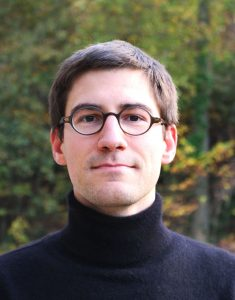Nicolas Curien

Nicolas Curien is Professor, since 2014, in the Mathematics Institute at Université Paris-Saclay, France. He specializes in probability theory and, more precisely, in random two-dimensional geometry. He received his PhD at Orsay (now Paris-Saclay) in 2011 and then spent two years at Sorbonne University as a CNRS researcher. His main focus is on the study of random discrete structures (such as random trees, random planar maps or graphs) and their scaling limits. He was awarded the Rollo-Davidson prize in 2015 and was a lecturer at the Saint-Flour summer school in 2019.

Nicolas will give this Doob Lecture at the World Congress in Seoul, in August. (See more about the Doob Lecture, honoring Joseph Leo Doob, below.)

## Random planar maps and Doob’s h-transformation

The $h$-transformation in the sense of Doob is now classic in probability theory and consists in twisting the transition probabilities $p(x,y)$ of a Markov process using a harmonic function $h(x)$ as follows:
$$q(x,y) := \frac{h(y)}{h(x)} p(x,y).$$
It can be used, for example, to quickly define processes conditioned on event of zero probability such as conditioning a Brownian motion to stay positive or conditioning an oscillating random walk to stay positive (Bertoin and Doney 1992). On the other hand, enumeration of planar maps (graphs drawn on the plane) is a well-established theory in combinatorics and in recent years it became apparent that some functions appearing in the asymptotic enumeration of maps (with a boundary) turned out to be harmonic functions for certain random walks on $\mathbb{Z}$. Using Markovian explorations of random planar maps known as the peeling processes (Angel 2003, Budd 2015) those functions can be used via $h$-transformations to define random planar maps conditioned on events of zero probability such as being infinite, having an infinite general or simple boundary or being of “hyperbolic” type.

In this talk, we will survey some of the recent developments in the construction and the study of infinite random planar maps using the peeling process and its connections with random walks.